Updating search results...

# 35 Results

View
Selected filters:
• NC.Math.6.G.1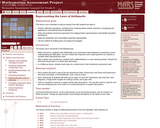Only Sharing Permitted
CC BY-NC-ND
Rating
0.0 stars

This lesson unit is intended to help you assess how well students are able to: Perform arithmetic operations, including those involving whole-number exponents, recognizing and applying the conventional order of operations; Write and evaluate numerical expressions from diagrammatic representations and be able to identify equivalent expressions; apply the distributive and commutative properties appropriately; and use the method for finding areas of compound rectangles.

Subject:
Geometry
Mathematics
Material Type:
Assessment
Lesson Plan
Provider:
Shell Center for Mathematical Education
Provider Set:
Mathematics Assessment Project (MAP)
06/24/2019Conditional Remix & Share Permitted
CC BY-NC
Rating
0.0 stars

Lesson OverviewStudents find the area of a parallelogram by rearranging it to form a rectangle. They find the area of a trapezoid by putting together two copies of it to form a parallelogram. By doing these activities and by analyzing the dimensions and areas of several examples of each figure, students develop and understand area formulas for parallelograms and trapezoids.Key ConceptsA parallelogram is a quadrilateral with two pairs of parallel sides. The base of a parallelogram can be any of the four sides. The height is the perpendicular distance from the base to the opposite side.A trapezoid is a quadrilateral with exactly one pair of parallel sides. The bases of a trapezoid are the parallel sides. The height is the perpendicular distance between the bases.You can cut a parallelogram into two pieces and reassemble them to form a rectangle. Because the area does not change, the area of the rectangle is the same as the area of the parallelogram. This gives the parallelogram area formula A = bh.You can put two identical trapezoids together to form a parallelogram with the same height as the trapezoid and a base length equal to the sum of the base lengths of the trapezoid. The area of the parallelogram is (b1 + b2)h, so the area of the trapezoid is one-half of this area. Thus, the trapezoid area formula is A = 12(b1 + b2)h.Goals and Learning ObjectivesDevelop and explore the formula for the area of a parallelogram.Develop and explore the formula for the area of a trapezoid.

Subject:
Geometry
Material Type:
Lesson Plan
Author:
Pearson
11/02/2020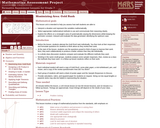Only Sharing Permitted
CC BY-NC-ND
Rating
0.0 stars

This lesson unit is intended to help you assess how well students are able to: Interpret a situation and represent the variables mathematically; select appropriate mathematical methods to use; explore the effects on the area of a rectangle of systematically varying the dimensions whilst keeping the perimeter constant; interpret and evaluate the data generated and identify the optimum case; and communicate their reasoning clearly.

Subject:
Geometry
Mathematics
Material Type:
Assessment
Lesson Plan
Provider:
Shell Center for Mathematical Education
Provider Set:
Mathematics Assessment Project (MAP)
06/24/2019Rating
0.0 stars

For this activity, students find the area of various triangles.

Subject:
Mathematics
Material Type:
Interactive
Provider:
Common Core Sheets
04/05/2017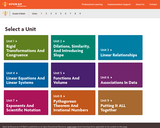Unrestricted Use
CC BY
Rating
0.0 stars

OER Initiative for mathematics

Subject:
Mathematics
Material Type:
Homework/Assignment
Author:
Multiple Authors
Creative Commons
10/23/2019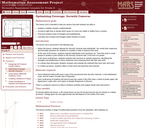Only Sharing Permitted
CC BY-NC-ND
Rating
0.0 stars

This lesson unit is intended to help sixth grade teachers assess how well students are able to: Analyze a realistic situation mathematically; construct sight lines to decide which areas of a room are visible or hidden from a camera; find and compare areas of triangles and quadrilaterals; and calculate and compare percentages and/or fractions of areas.

Subject:
Geometry
Mathematics
Material Type:
Assessment
Lesson Plan
Provider:
Shell Center for Mathematical Education
Provider Set:
Mathematics Assessment Project (MAP)
06/24/2019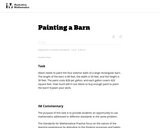Unrestricted Use
CC BY
Rating
0.0 stars

The purpose of this task is to provide students an opportunity to use mathematics addressed in different standards in the same problem.

Subject:
Mathematics
Material Type:
Activity/Lab
Provider:
Illustrative Mathematics
Provider Set:
Illustrative Mathematics
Author:
Illustrative Mathematics
05/01/2012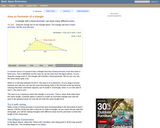Rating
0.0 stars

An interactive applet and associated web page that show the relationship between the perimeter and area of a triangle. It shows that a triangle with a constant perimeter does NOT have a constant area. The applet has a triangle with one vertex draggable and a constant perimeter. As you drag the vertex, it is clear that the area varies, even though the perimeter is constant. Optionally, you can see the path traced by the dragged vertex and see that it forms an ellipse. A link takes you to a page where this effect is exploited to construct an ellipse with string and pins. The applet can be enlarged to full screen size for use with a classroom projector. This resource is a component of the Math Open Reference Interactive Geometry textbook project at http://www.mathopenref.com.

Subject:
Geometry
Mathematics
Material Type:
Simulation
Provider:
Math Open Reference
Author:
John Page
05/07/2019Conditional Remix & Share Permitted
CC BY-NC-SA
Rating
0.0 stars

This course was created by the Rethink Education Content Development Team in partnership with the North Carolina Virtual Public Schools. This course is aligned to the NC Standards for 6th Grade Math.&nbsp;

Subject:
Mathematics
Material Type:
Activity/Lab
Assessment
Formative Assessment
Full Course
Homework/Assignment
Lecture Notes
Presentation
Vocabulary
Author:
AMBER GARVEY
08/25/2022Conditional Remix & Share Permitted
CC BY-NC-SA
Rating
0.0 stars

This course was created by the Rethink Education Content Development Team in partnership with the North Carolina Virtual Public Schools. This course is aligned to the NC Standards for 6th Grade Math.&nbsp;

Subject:
Mathematics
Material Type:
Full Course
Author:
AMBER GARVEY
11/30/2021Conditional Remix & Share Permitted
CC BY-NC-SA
Rating
0.0 stars

This course was created by the Rethink Education Content Development Team in partnership with the North Carolina Virtual Public Schools. This course is aligned to the NC Standards for 6th Grade Math. &nbsp;

Subject:
Mathematics
Material Type:
Activity/Lab
Assessment
Formative Assessment
Full Course
Homework/Assignment
Lesson
Module
Vocabulary
Author:
AMBER GARVEY
03/08/2022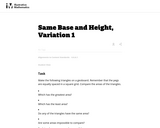Unrestricted Use
CC BY
Rating
0.0 stars

This is the first version of a task asking students to find the areas of triangles that have the same base and height, and is the most concrete.

Subject:
Mathematics
Material Type:
Activity/Lab
Provider:
Illustrative Mathematics
Provider Set:
Illustrative Mathematics
Author:
Illustrative Mathematics
05/01/2012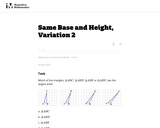Unrestricted Use
CC BY
Rating
0.0 stars

This is the second version of a task asking students to find the areas of triangles that have the same base and height. This presentation is more abstract as students are not using physical models.

Subject:
Mathematics
Material Type:
Activity/Lab
Provider:
Illustrative Mathematics
Provider Set:
Illustrative Mathematics
Author:
Illustrative Mathematics
05/01/2012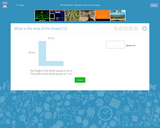Rating
0.0 stars

For this activity, students calculate the area of different shapes.

Subject:
Mathematics
Material Type:
Interactive
Provider:
Doctor Genius
04/18/2017